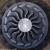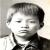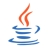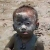定时(每隔数十毫米)执行有哪些方法

IdleMan 发布于 2012/06/25 22:26

年薪达不到25W大数据工程师、拿不到Offer全额退款->>>1.间隔时间为毫秒级

2.运行稳定

3.资源占用少

01.间隔时间为毫秒级0sleep塞住指定毫秒。sleep会把程序挂起，所谓挂起就是让线程休眠，所谓休眠就是让操作系统暂时不理会这个线程，这段休眠的时间CPU基本上认为没有这个线程存在。
00#define GET_CPU_CLK(re) do { \
unsigned int __a,__d; \
__asm__ __volatile__("rdtsc" : "=a" (__a), "=d" (__d)); \
(re) = ((unsigned long)__a) | (((unsigned long)__d)<<32); \
} while(0)
#define CPU_CLK_START() GET_CPU_CLK(s_last_count)
#define CPU_CLK_END(re) do{GET_CPU_CLK(re); (re) =(re) - s_last_count;	}while(0)
#define CPU_CLK_2_TIME(re,mode) do{\
const long MODE_CLK = {1000,1000000};\
CPU_CLK_START();\
sleep(1);\
CPU_CLK_END(s_last_count);\
re = (re * MODE_CLK[((mode) != 0)]) /  s_last_count;\
}while(0)
static unsigned long long  s_last_count = 0;

unsigned long long _clk;
unsigned long long _total_clk;
void test(void){
...
}

void main_test(void){
int i;
for (i = 0 ; i < 10 ; i++){
CPU_CLK_START();
test();
CPU_CLK_END(_clk);
_total_clk += _clk;
}
CPU_CLK_2_TIME(_total_clk,1); // count us; if count ms , used CPU_CLK_2_TIME(var,0);
printf("total times is %lld(us)!\n",_total_clk);
}

0